Math resources Algebra

Number patterns

# Number patterns

Here you will learn about number patterns, including how to find and extend rules for sequences, input/output tables and shape patterns.

Students will first learn about number patterns as part of operations and algebraic thinking in 4th and 5th grade. They continue to build on this knowledge in middle school and high school.

## What are number patterns?

Number patterns are groups of numbers that follow rules. They can use input/output tables to create sequences.

Two types of sequences are arithmetic and geometric.

• An arithmetic sequence is a list of numbers where the same amount is either being added or subtracted every time.

Each sequence has a starting number, a rule and terms (the numbers that make up the sequence).

For example,

Rule: Subtract 6 each time.

There are also patterns, between the terms of two or more arithmetic sequences.

For example,

Both sequences start at 0. If you multiply the left column by 3, you get the terms in the right column.

This is because using the rule +9 is three times more than the rule +3.

• A geometric sequence is a number pattern where the rule is multiplication or division.

For example,

Rule: Multiply the previous term by 5.

For example,

Rule: Divide the previous term by 3.

Step-by-step guide: Sequences

• Input/output tables are tables that are used to show two sets of numbers that are related by a rule. The rule can be one step or multi-step, but has to work for each relationship shown in the table.

For example,

What is the rule for the table below?

To find the rule, look for the relationship between the input and the corresponding output.

Notice, 11 is being added to each input to get the output, so the rule is ‘add 11.

Step-by-step guide: Input/output tables

As students learn to work with number patterns, they can learn more about generalizing patterns by working with shape patterns.

• Shape patterns are any set of polygons, 3D shapes, letters or symbols that follow non-operational rules. There are repeating shape patterns and growing shape patterns.

A repeating pattern has a core that repeats over and over again.

For example,

The core of the pattern above is:

The core can be used to extend the pattern.

The next shape in the pattern would be: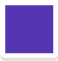because the last part of the core shown is:.

• A growing pattern has parts that stay the same, but other parts that change.

For example,

• Changing – the left column starts with 0 and increases by 2.
• Staying the same – the 1 cube on the top right.

Step-by-step guide: Shape patterns

### What are number patterns?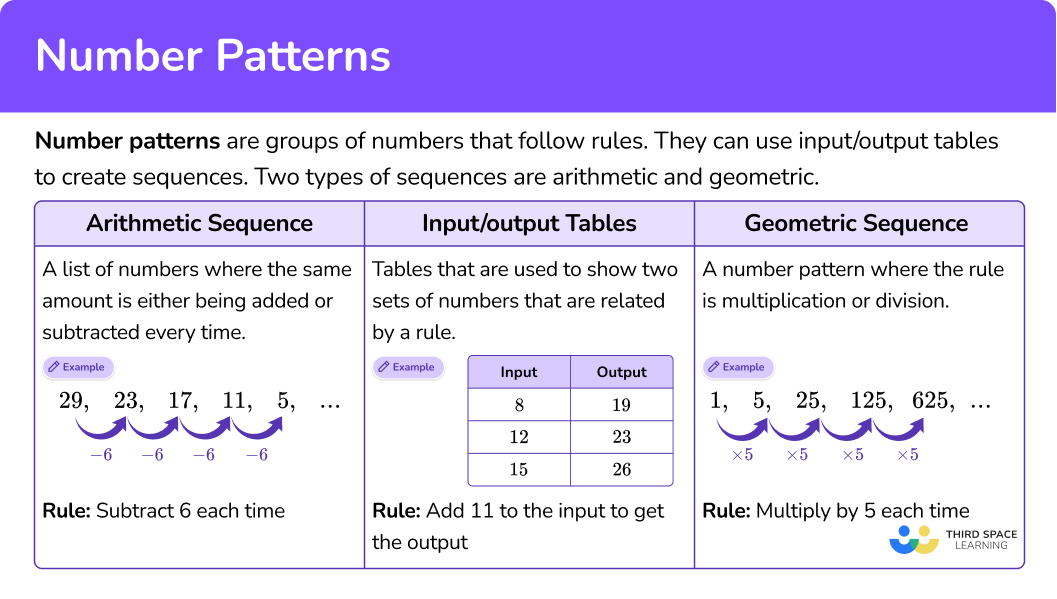## Common Core State Standards

How does this relate to 4th grade math and 5th grade math?

• Grade 4 – Operations and Algebraic Thinking (4.OA.C.5)
Generate a number or shape pattern that follows a given rule. Identify apparent features of the pattern that were not explicit in the rule itself.

For example, given the rule “Add 3 ” and the starting number 1, generate terms in the resulting sequence and observe that the terms appear to alternate between odd and even numbers. Explain informally why the numbers will continue to alternate in this way.

• Grade 5 – Operations and Algebraic Thinking (5.OA.B.3)
Generate two numerical patterns using two given rules. Identify apparent relationships between corresponding terms. Form ordered pairs consisting of corresponding terms from the two patterns, and graph the ordered pairs on a coordinate plane.

For example, given the rule “Add 3 ” and the starting number 0, and given the rule “Add 6 ” and the starting number 0, generate terms in the resulting sequences, and observe that the terms in one sequence are twice the corresponding terms in the other sequence. Explain informally why this is so.

## How to use number patterns

There are a lot of ways to use number patterns. For more specific step-by-step guides, check out the pages linked in the “What are number patterns?” section above or read through the examples below.

## Number patterns examples

### Example 1: geometric sequence

What are the next three terms in the pattern?

3, 6, 12, 24, 48…

1. Identify the rule.

Rule: Multiply the previous term by 2.

2Use the rule to extend the pattern.

Multiply the last term by 2 to extend the pattern to the next three terms.

3State and explain any patterns within the terms.

Except for the first term, all the terms are even.

The first term is odd, but multiplying it by 2 (even), makes the next term even.

From then on it is always an even term times 2 \rightarrow \text { even } \times \text { even }=\text { even }.

### Example 2: compare arithmetic sequences

Compare the following sequences:

0, 2, 4, 6, 8… \, and \, 0, 12, 24, 36, 48…

Identify the rule of each sequence.

Find a pattern between the related terms of each sequence.

Use the pattern to write a comparison statement.

### Example 3: input/output table – identify the rule

What is the rule for the table?

Look at the relationship between the input and the corresponding output.

Decide if the rule is add/subtract or multiply/divide.

Write the rule.

### Example 4: input/output table – find a missing value

Find the missing value in the table.

Look at the relationship between the input and the corresponding output.

Decide if the rule is add/subtract or multiply/divide.

Write the rule.

Use the rule to find the missing value(s).

### Example 5: 3D shape pattern, repeating

Create a rule for the pattern and find the next shape.

Identify the core – the part of the pattern that repeats.

Use the core to find and justify the next part in the pattern.

### Example 6: shape pattern, growing

Create a rule for the pattern and find the next shape.

Identify what is changing and what is staying the same.

Create a rule based on Step 1.

Use the rule to find and justify the next part in the pattern.

### Teaching tips for number patterns

• There are many printable number patterns worksheets that can be used, but also give students the opportunity to create their own patterns. Then let their classmates find the rules and extend them.

This helps keep students interested and deepens their understanding of patterns by challenging them to create and giving them opportunities to engage in discourse around patterns.

• Support students who are not yet confident in all operations by providing useful tools such as number lines, hundreds boards or counters. Completing calculations should not be a burden when working with patterns.

• Focusing on justifying pattern rules is as important as identifying them. Justification is not only a necessary mathematical practice, but doing it can teach students how to write better rules.

Thinking about why a rule works, draws attention to the general parts of a pattern – how they are changing or staying the same, which is the basis of a valid mathematical generalization.

### Easy mistakes to make

• Writing a rule for a sequence that is not term to term
Work with sequences in elementary school is recursive. Therefore, it is important to focus on what is happening from term to term in order to define the rule. This can be confusing when an arithmetic sequence is a list of multiples.

For example,
What is the rule for the pattern 10, 20, 30, 40, 50?

To find the next term, you need to add 10, not multiply.
Even though the terms are multiples of 10, for this to be the rule, there would need to be corresponding inputs and outputs, such as…

• Using only the first number and second number to create the rule
Often by the third number the pattern can be identified, but it is still important to look at all of the numbers in a pattern before finalizing the rule. Looking at just too few can lead to the incorrect rule.

For example,
What is the rule for the pattern 3, 6, 12, 24, 28?

• Making an operational error
Since finding the rule and extending the pattern require a calculation, this leaves room for mistakes to be made. Always double check your work and take time to think about if your answers are reasonable.

• Thinking there is only one way to write a growing shape pattern rule
Often there is more than one way to describe what is changing and what is staying the same in a growing shape pattern. Encourage students to look for more than one way to describe their rule, as this is a helpful stepping stone to equivalent expressions.

### Practice number patterns questions

1. What is the next number in the pattern?

4, 20, 100, 500, 2,500…

3,0005005,00012,500Identify the rule.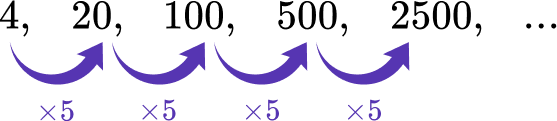Rule: Multiply the previous term by 5.

Multiply the last term by 5 to extend the pattern.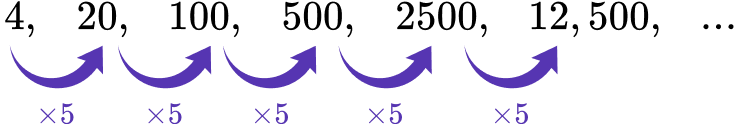2. Starting number: 68

Rule: subtract 6 each time.

Which statement is true about the terms of the sequence described above?

All the terms are evenAll the terms are oddThe terms alternate between even and oddThe first term is even and the rest are oddExtend the sequence based on the starting number and the rule.Since 6 (even number) is being subtracted each time, and even even = even, all the terms are even.

3. What is the rule for the table?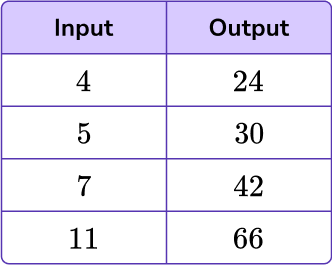Multiply the input by 6Multiply the input by 8Looking from each input to output, see if you notice an obvious relationship.

If not, use subtraction to find the difference between each input and the corresponding output.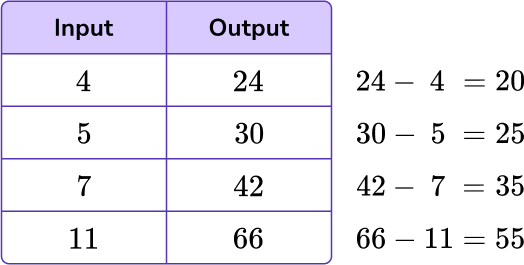From each input to output, the difference is not the same, so the rule is not addition or subtraction.

Try to find a relationship that involves multiplying or dividing.

Since the relationship from input to output is increasing, try multiplication.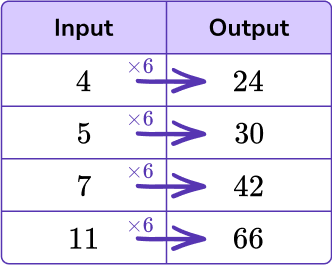Rule: Multiply the input by 6.

4. Find the missing value in the table.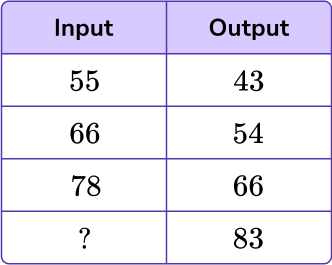94719572Looking from each input to output, see if you notice an obvious relationship.

If not, use subtraction to find the difference between each input and the corresponding output.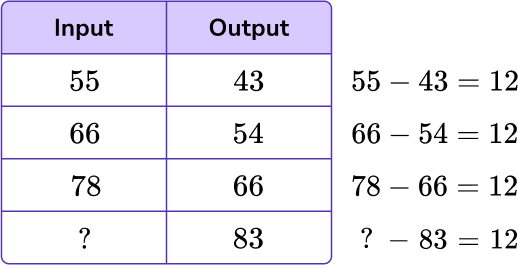Each output is 12 less than the input, so the rule is subtraction.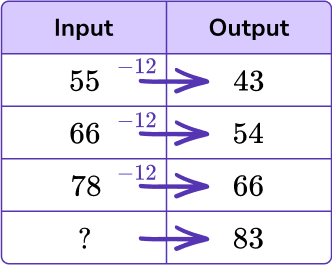Rule: Subtract 12 from the input.

Since the input is missing, thinking about what number subtracted by 12 will equal 83 :

?-12=83

95-12=83

The missing value in the table is 95.

5. What is the next shape in the pattern?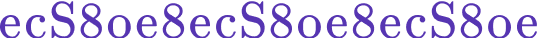c8SoThis pattern goes e, c, S, 8, o, e, 8 and then repeats.6. What is the next part in the pattern?Identify what is changing and what is staying the same:

• Changing – one purple in the middle and one green on either side, two purple in the middle and two green on either side, three purple in the middle and three green on either side.
• Staying the same – There is always a purple x with a green x beside it and below it at the top.

Rule: Start with a purple x with a green x to the right and below it. Repeat, adding the 1 st term again, but to the left and down one .

The next part in the pattern is: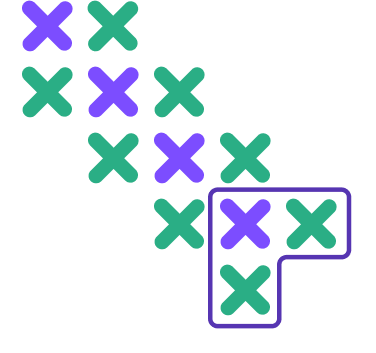because it adds the first term again, but to the left and down one (shown in purple outline).

## Number patterns FAQs

Do the terms of a sequence always have to be whole numbers?

No, since students are most familiar with whole number operations, the standards start with these numbers. However, in middle school, sequences grow to include integers and other types of rational numbers. This also continues in later grades to include sequences with complex numbers.

What are some well known sequences?

Fibonacci’s sequence (or fibonacci numbers) and triangular numbers (pascal’s triangle) are two sequences that are commonly explored in upper level mathematics.

Can shape patterns include fractions and decimals?

While shape patterns do not typically include fractions or decimals, they could be used in a way that does not involve calculations (for example in the repeating pattern \, \cfrac{1}{5} \, , \, \cfrac{1}{8} \, , \, \cfrac{1}{5} \, , \, \cfrac{1}{8} \, , \, \cfrac{1}{5} \, , \, \cfrac{1}{8} \, … ).

Are there other types of sequences besides arithmetic and geometric?

Yes, there are sequences that involve square numbers, cube numbers, and other more complex operations.

• Angles
• 2D shapes
• Lines
• Triangles

## Still stuck?

At Third Space Learning, we specialize in helping teachers and school leaders to provide personalized math support for more of their students through high-quality, online one-on-one math tutoring delivered by subject experts.

Each week, our tutors support thousands of students who are at risk of not meeting their grade-level expectations, and help accelerate their progress and boost their confidence.